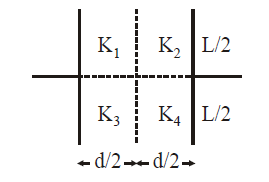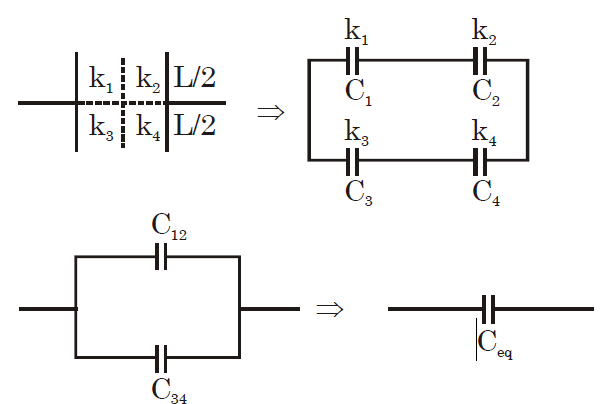# Q. A parallel plate capacitor with square plates is filled with four dielectrics of dielectric constants $K_1, K_2, K_3, K_4$ arranged as shown in the figure. The effective dielectric constant K will be :JEE Main JEE Main 2019

Solution:

## $C_{12} = \dfrac{C_{1} C_{2}}{C_{1 }+ C_{2}} = \dfrac{\dfrac{k_{1} \in_{0} \dfrac{L}{2} \times L }{d/2} . \dfrac{k_{2} \left[\in_{0} \dfrac{L}{2} \times L\right]}{d/2}}{\left(k_{1} +k_{2}\right) \left[\dfrac{\in_{0} . \dfrac{L}{2} \times L}{d/2}\right]}$ $C_{12} = \dfrac{k_{1}k_{2}}{k_{ 1} +k_{2}} \dfrac{\in_{0} L^{2}}{d}$ in the same way we get, $C_{34} = \dfrac{k_{3}k_{4} }{k_{3} +k_{4}} \dfrac{ \in_{0} L^{2}}{d}$ $\therefore C_{eq} = C_{12} + C_{34} = \left[\dfrac{k_{1}k_{2}}{k_{1} + k_{2}} + \dfrac{k_{3}k_{4}}{k_{3} + k_{4}} \right] \dfrac{\in_{0} L^{2}}{d}$ ....(i) Now if $k_{eq} = k , C_{eq} = \dfrac{k \in_{0} L^{2}}{d}$ .....(ii) on comparing equation (i) to equation (ii), we get $k_{eq} = \dfrac{k_{1}k_{2} \left(k_{3} +k_{4}\right)+k_{3} k_{4} \left(k_{1 }+k_{2}\right)}{\left(k_{1} +k_{2} \right)\left(k_{3}+ k_{4}\right)}$ This does not match with any of the options so probably they have assumed the wrong combination $C_{13} = \dfrac{k_{1} \in_{0} L \dfrac{L}{2}}{d/2} + k_{3} \in_{0} \dfrac{L. \dfrac{L}{2}}{d/2}$ $= \left(k_{1} + k_{3}\right) \dfrac{\in_{0} L^{2}}{d}$ $C_{24} = \left(k_{2 } + k_{4}\right) \dfrac{\in_{0} L^{2}}{d}$ $C_{eq} = \dfrac{C_{13} C_{24}}{C_{13} C_{24}} = \dfrac{\left(k_{1} + k_{3} \right)\left(k_{2} +k_{4}\right) }{\left(k_{1} + k_{2} + k_{3} + k_{4}\right)} \dfrac{\in _{0} L^{2}}{d}$ $= \dfrac{k \in_{0}L^{2}}{d}$ $k = \dfrac{\left(k_{1} +k_{3}\right)\left(k_{2} +k_{4}\right)}{\left(k_{1} +k_{2 }+ k_{3} + k_{4}\right) }$ However this is one of the four options. It must be a "Bonus" logically but of the given options probably they might go with (4)You must select option to get answer and solution

## 1. The increase in pressure required to decrease the 200 L volume of a liquid by 0.004% (in kPa) is (Bulk modulus of the liquid ==2100 MPa)

Maharashtra CET 2006 Mechanical Properties Of Solids

## 2. To have large selectivity in a series LCR circuit

COMEDK 2006 Alternating Current

## 3. The electric field and the potential of an electric dipole vary with distance r as

Manipal 1980 Electric Charges And Fields

## 4. To draw maximum current from a combination of cells, how should the cells be grouped?

AFMC 2006 Current Electricity

NEET 2019 Waves

AFMC 2010 Atoms

## 7. The period of revolution of planet A around the sun is 8 times that of B. The distance of A from the sun is how many times greater than that of B from the sun?

AIPMT 1997 Gravitation

## 8. The temperature of 100 g of water is to be raised from 24°C to 90°C by adding steam to it. The mass of the steam required for this purpose is

JIPMER 2015 Thermal Properties Of Matter

## 9. Two discs of same moment of inertia rotating about their regular axis passing through centre and perpendicular to the plane of disc with angular velocities $\omega_1$ and $\omega_2$ . They are brought into contact face to face coinciding the axis of rotation. The expression for loss of energy during this process is:-

NEET 2017 System Of Particles And Rotational Motion

## 10. The hot wire ammeter measures

Rajasthan PMT 2004 Alternating Current